# Trinomial Tree for Pricing European Options

Binomial trees are often used to price options that have no closed-form analytical solutions. However, they can easily become large and inefficient to implement. Trinomial trees, however, are more efficient and converge more rapidly than their binomial counterparts. Moreover, trinomial trees are only slightly more complex to implement than binomial trees.

The VBA for trinomial pricing lattice is described by this pseudocode

1. Calculate the jump sizes (u, d)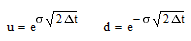2. Calculate the probabilities (pu, pm, pd)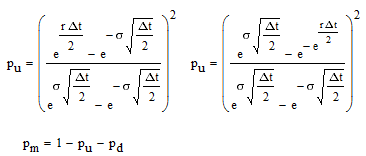3. Create a tree of share prices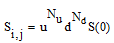4. Calculate the payoff at maturity at the final node
5. Create the option price lattice through backwards induction. Step back through the lattice to the initial node.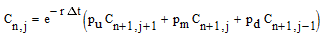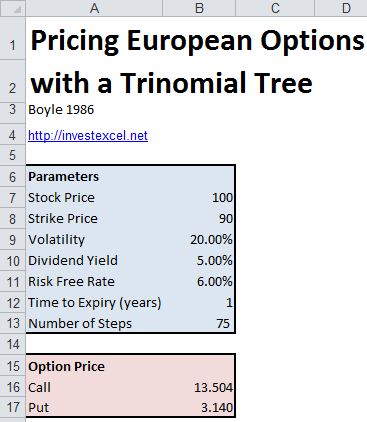This pseudocode is implemented in the following VBA function.

```Function EuropeanOption(OptionType As String, S As Double, X As Double, T As Double, r As Double, div As Double, vol As Double, nSteps As Integer)   Dim C() As Double Dim u As Double, d As Double Dim Pu As Double, Pd As Double, Pm As Double, cc As Double Dim dt As Double, Df As Double, St As Double Dim i As Integer, j As Integer, cp As Integer, k As Integer   dt = T / nSteps cc = r - div   ReDim C(nSteps * 2 + 1)   'Option Type If OptionType = "Call" Then cp = 1 ElseIf OptionType = "Put" Then cp = -1 End If   'Jump Sizes u = Exp(vol * Sqr(2 * dt)) d = Exp(-vol * Sqr(2 * dt))   'Transition Probabilities Pu = ((Exp(cc * dt / 2) - Exp(-vol * Sqr(dt / 2))) / (Exp(vol * Sqr(dt / 2)) - Exp(-vol * Sqr(dt / 2)))) ^ 2 Pd = ((Exp(vol * Sqr(dt / 2)) - Exp(cc * dt / 2)) / (Exp(vol * Sqr(dt / 2)) - Exp(-vol * Sqr(dt / 2)))) ^ 2 Pm = 1 - Pu - Pd   'Share Price Tree (Forward Tree) For i = 0 To (2 * nSteps) C(i) = WorksheetFunction.Max(0, cp * (S * u ^ WorksheetFunction.Max(i - nSteps, 0) * d ^ WorksheetFunction.Max(nSteps - i, 0) - X)) Next   'Calculate Option Price at Maturity (Backwards Induction) Df = Exp(-r * dt) For k = nSteps - 1 To 0 Step -1 For i = 0 To (k * 2) C(i) = (Pu * C(i + 2) + Pm * C(i + 1) + Pd * C(i)) * Df Next Next   'Option Price EuropeanOption = C(0)   End Function```

This Excel spreadsheet implements the VBA given above (this spreadsheet prices American options with a trinomial tree).